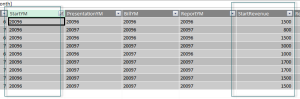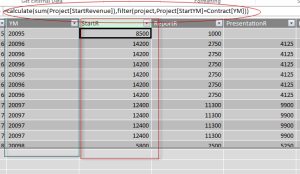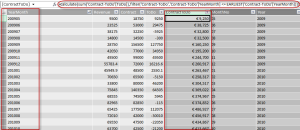﻿﻿ Powerful DAX CALCULATE() Function - CloudFronts

# Powerful DAX CALCULATE() Function

Posted On June 24, 2015 by Admin Posted in  Tagged in ,

The CALCULATE function in DAX is the magic key for many calculations we can do in PowerPivot.

Below is the syntax:

CALCULATE( <expression>, <filter1>, <filter2>… )

The expression that we put in the first parameter has to be evaluated to return the result (i.e. a value, not a table). For this reason, the expression is usually an aggregation function like SUM, MIN, MAX, COUNTROWS and so on.

This expression is evaluated in a context that is modified by the filters. A key point is that these filters can both enlarge and restrict the current context of evaluation. Let’s try to understand what it means by considering a few examples.

The following data model we have imported in PowerPivot named ‘Contract’ & ‘Project’

Scenario 1
Compare Contract & Project data model on YearMonth Column and take sum of multiple records of revenue column of Project data model into Contract data model
Project data model has StartYM & StartRevenue Column as shown belowAnd Contract data model has YM column, using Project data model StartYM, StartRevenue columns & Contract data model YM column, here we have derived StartR column with the help of Calculate() DAX function as shown belowFormula is
=calculate(sum(Project[StartRevenue]),filter(project,Project[StartYM]=Contract[YM]))
Scenario 2
Calculate running total of ToDo column in ‘Contract-ToDo’ data model on basis of YearMonth column as shown belowFormula is
=calculate(sum(‘Contract-ToDo'[ToDo]),filter(‘Contract-ToDo’,’Contract-ToDo'[YearMonth] <= EARLIEST('Contract-ToDo'[YearMonth])))